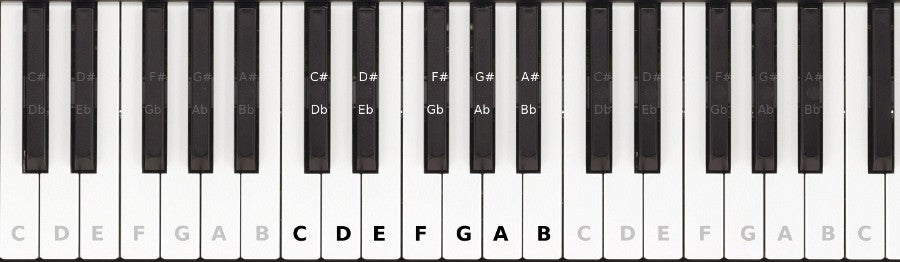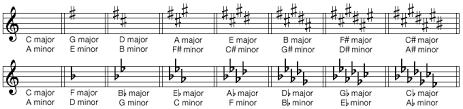# Circle of Fifths

The Circle of FifthsThe Circle of Fifths organizes all of the Keys and their respective Key Signatures. The keys on the right hand side of the circle are the sharp keys while the keys on the left hand side are the flat keys. As we go around the circle clockwise, we go up a fifth to each key, therefore, going counterclockwise, we go down a fifth.

To count up a fifth, the note we start on is 1, going up we count each letter, then note number 5 is the fifth.

Here's an example...

We start with C, so C is 1. Then, D is 2, E, is 3, F is 4, and G is 5. So, a fifth above C is G.

Another example...
G A B C D
1 2 3 4 5
so a fifth above G is D.

One more example...
D E F G A
1 2 3 4 5
so a fifth above D is A.

When playing the piano, a fifth is really easy to find. When you put your fingers each on their own key without skipping any keys, the keys the thumb and the pinky are on are a fifth apart.Now, let's work out the sharp keys on the right side of the circle.

We start with the key of C on the top. C Major has no sharps and no flats.So the first sharp key is up a fifth, G. Adding sharps follows a pattern. For each new key, we keep the sharps we already have and then add a sharp to the 7th note of the scale. (The easier way to think about the 7th, is that it is the note below the name.)

For the key of G, we add a sharp on F. So we just have one sharp, F.A fifth above G is D (Click here if you need a reminder of how to figure that out.)
For the key of D, we add a sharp on C. Now we have two sharps, F C.A fifth above D is A.
For the key of A, we add a sharp on G. Now we have three sharps, F C G.A fifth above A is E.
For the key of E, we add a sharp on D. Now we have four sharps, F C G D.A fifth above E is B.
For the key of B, we add a sharp on A. Now we have five sharps, F C G D A.And so on, eventually, we end up with 7 sharps. If you have followed the pattern, you will know that the sharps have a specific order.

F C G D A E B

A sentence to help us remember that order...

Fred Copies Grace's Diary After Every Bedtime
(A student came up with that for me years and years ago, and I have used it ever since.)